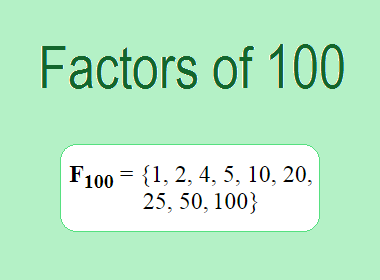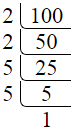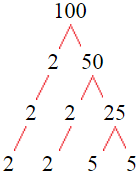# Factors of 100The factors of 100 are 1, 2, 4, 5, 10, 20, 25, 50, and 100 i.e. F100 = {1, 2, 4, 5, 10, 20, 25, 50, 100}. The factors of 100 are all the numbers that can divide 100 without leaving a remainder.

We can check if these numbers are factors of 100 by dividing 100 by each of them. If the result is a whole number, then the number is a factor of 100. Let's do this for each of the numbers listed above:

·        1 is a factor of 100 because 100 divided by 1 is 100.

·        2 is a factor of 100 because 100 divided by 2 is 50.

·        4 is a factor of 100 because 100 divided by 4 is 25.

·        5 is a factor of 100 because 100 divided by 5 is 20.

·        10 is a factor of 100 because 100 divided by 10 is 10.

·        20 is a factor of 100 because 100 divided by 20 is 5.

·        25 is a factor of 100 because 100 divided by 25 is 4.

·        50 is a factor of 100 because 100 divided by 50 is 2.

·        100 is a factor of 100 because 100 divided by 100 is 1.

## How to Find Factors of 100?

1 and the number itself are the factors of every number. So, 1 and 100 are two factors of 100. To find the other factors of 100, we can start by dividing 100 by the numbers between 1 and 100. If we divide 100 by 2, we get a remainder of 0. Therefore, 2 is a factor of 100. If we divide 100 by 3, we get a remainder of 1. Therefore, 3 is not a factor of 100.

Next, we can check if 4 is a factor of 100. If we divide 100 by 4, we get a remainder of 0. Therefore, 4 is also a factor of 100. We can continue this process for all the possible factors of 100.

Through this process, we can find that the factors of 100 are 1, 2, 4, 5, 10, 20, 25, 50, and 100. These are the only numbers that can divide 100 without leaving a remainder.

********************

********************

## Properties of the Factors of 100

The factors of 100 have some interesting properties. One of the properties is that the sum of the factors of 100 is equal to 217. We can see this by adding all the factors of 100 together:

1 + 2 + 4 + 5 + 10 + 20 + 25 + 50 + 100 = 217

Another property of the factors of 100 is that the prime factors of 100 are 2, and 5 only.

## Applications of the Factors of 100

The factors of 100 have several applications in mathematics. One of the applications is in finding the highest common factor (HCF) of two or more numbers. The HCF is the largest factor that two or more numbers have in common. For example, to find the HCF of 100 and 150, we need to find the factors of both numbers and identify the largest factor they have in common. The factors of 100 are 1, 2, 4, 5, 10, 20, 25, 50, and 100. The factors of 150 are 1, 2, 3, 5, 6, 10, 15, 25, 30, 50, 75, and 150. The largest factor that they have in common is 50. Therefore, the HCF of 100 and 150 is 50.

Another application of the factors of 100 is in prime factorization. Prime factorization is the process of expressing a number as the product of its prime factors. The prime factors of 100 are 2, and 5 since these are the only prime numbers that can divide 100 without leaving a remainder. Therefore, we can express 100 as:

100 = 2 × 2 × 5 × 5

We can do prime factorization by division and factor tree method also. Here is the prime factorization of 100 by division method,100 = 2 × 2 × 5 × 5

Here is the prime factorization of 100 by the factor tree method,100 = 2 × 2 × 5 × 5

## Conclusion

The factors of 100 are the numbers that can divide 100 without leaving a remainder. The factors of 100 are 1, 2, 4, 5, 10, 20, 25, 50, and 100. The factors of 100 have some interesting properties, such as having a sum of 217. The factors of 100 have several applications in mathematics, such as finding the highest common factor and prime factorization.